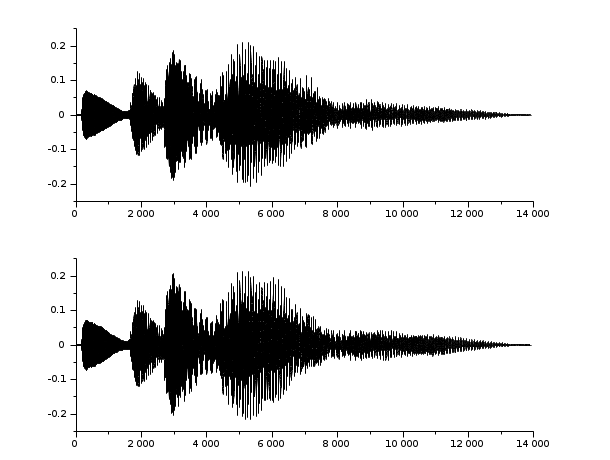Scilab Home page | Wiki | Bug tracker | Forge | Mailing list archives | ATOMS | File exchange
Change language to: English - Français - Português - Русский

See the recommended documentation of this function

Scilab help >> Sound file handling > wavread

Calling Sequence

```y=wavread(wavfile)

Arguments

wavfile

string (The .wav extension is appended if no extension is given)

Fs

integer, frequency sampling in Hz (number of samples per second).

ext

string (`'size'`) or string('info') or integer (to read n samples) or 1 x 2 integer vector [n1,n2] (to read from n1 to n2).

Description

Utility function to read `.wav` sound file. `wavread(wavfile)` loads a sound file specified by the string wavfile, returning the sampled data in y. Amplitude values are in the range [-1,+1]. Supports multi-channel data in the following formats: 8-bit mu-law, 8-, 16-, and 32-bit linear, and floating point.

`[y,Fs,bits]=wavread(wavfile)` returns the sample rate (Fs) in Hertz and the number of bits per sample used to encode the data in the file.

`wavread(wavfile,n)` returns the first n samples from each channel.

`wavread(wavfile,[n1,n2])` returns samples n1 to n2.

`wavread(wavfile,'size')` returns the size of the audio data contained in the file in place of the actual audio data, returning the vector as [channels samples].

`wavread(wavfile,'info')` returns information about the audio data contained in the file in place of the actual audio data, returning the vector as [data format, number of channels, samples per second per channel, estimate of bytes per second needed, byte alignment of a basic sample block, bits per sample, length of sound data in bytes, bytes per sample (per channel)].

Examples

```wavread("SCI/modules/sound/demos/chimes.wav","size")
subplot(2,1,1)
plot2d(y(1,:)) // first channel
subplot(2,1,2)
plot2d(y(2,:)) // second channel
y=wavread("SCI/modules/sound/demos/chimes.wav",[1 5]) //the first five samples```